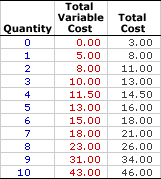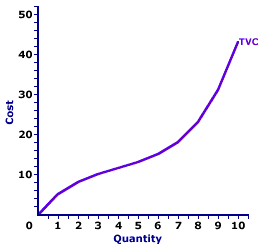Wednesday  September 27, 2023
 AmosWEB means Economics with a Touch of Whimsy!REAL INTEREST RATE: The market, or nominal interest rate, after adjusting for inflation. This is the interest rate lenders receive and borrowers pay expressed in real dollars. There two ways to think about the real interest rate, (1) the historical, after-the-fact, interest rate and (2) the desired interest rate lenders and borrowers have in mind when entering into a loan. The first one tells us the purchasing power of any interest payments received or paid. The second way of looking at the real interest rate is based on expectations of the future.TOTAL VARIABLE COST:

Cost of production that does change with changes in the quantity of output produced by a firm in the short run. Total variable cost is one part of total cost. The other is total fixed cost. Variable cost depends on the level of output. If a firm produces more output, then variable cost is greater. If a firm produces no output, then variable cost is zero. A cost measure directly related to total variable cost is average variable cost.
Total variable cost is the opportunity cost incurred in the short-run production that depends on the quantity of output. As the name clearly implies, total variable cost is variable, it changes. If a firm produces a little output, then total variable cost is less. If a firm produces a lot of output, then total variable cost is more.

A firm can avoid variable cost in the short run by reducing production to zero. This bit of information often comes in handy when the price received by a firm is so low that it falls short off covering variable cost at any positive quantity of production. As such, the firm might find it most "profitable" to cut losses by shutting down production and avoiding variable cost, until the price increases.

### Variable Inputs

Total variable cost is usually, not always but usually, associated with inputs that are variable in the short run. For example, The Wacky Willy Company operates in the short run with labor (the workers) as a variable input and capital (the building and equipment) as a fixed input. The cost associated with labor is a prime candidate to be a variable cost. This includes hourly wage payments to the workers and any hourly fringe benefits paid on behalf of the workers.

While labor is usually isolated as THE variable input in the short run, most short-run production has other variable inputs, too. The Wacky Willy Company uses an assortment of other variable inputs, all of which are part of variable cost--including cuddly cloth, squeezeably soft stuffing, and thread that make up the Stuffed Amigos; electricity needed to run the machinery; and boxes, plastic wrapping, and other packing materials used to ship the Stuffed Amigos to customers. When The Wacky Willy Company makes more Stuffed Amigos, they incur a greater cost for these variable inputs.

### The Cost of Stuffed Amigos

Total Variable CostThe table to the right summarizes the total variable cost of producing Wacky Willy Stuffed Amigos. The left-hand column is the quantity of Stuffed Amigos coming off the assembly line each minute, ranging from 0 to 10. For reference, the right-hand column is the total cost of producing Stuffed Amigos, the combination of total variable and total fixed cost.

The center column is the total variable cost of producing each quantity, which is exactly \$0 if no Stuffed Amigos are produced and rises to \$43 if 10 Stuffed Amigos are produced. This is the essence of variable cost. Greater production entails greater total variable cost.

• The most obvious point is that total variable cost increases with increased production. Producing more Stuffed Amigos means higher total variable cost. This makes sense. To produce more Stuffed Amigos, The Wacky Willy Company needs to hire more labor and buy more materials. Because these inputs incur a cost, total variable cost rises with extra production.

• Moreover, total variable cost is zero when no Stuffed Amigos are produced. The Wacky Willy Company incurs no variable cost when they employ no workers and buy no materials--their variable inputs.

• A last note is that the incremental increase in total variable cost is NOT the same for each quantity. In other words, total variable cost does not rise at a constant rate. This incremental change in total variable cost reflects marginal cost, a key concept in the short-run production and supply decision of a firm.

### The Total Variable Cost Curve

Total Variable Cost CurveThe total variable cost curve graphically represents the relation between total variable cost incurred by a firm in the short-run product of a good or service and the quantity produced. The total variable cost curve (TVC) for Wacky Willy Stuffed Amigos production is illustrated in the graph to the right. Because total variable cost increases with the quantity produced, the total variable cost curve has a positive slope.

The most striking feature of the total variable cost curve is its shape. The total variable cost curve emerges from the origin, then twists and turns its way to \$43. This curve begins relatively steep, then flattens, before turning increasingly steep once again.

The slope of the total variable cost curve flattens as the first four Stuffed Amigos are produced due to increasing marginal returns found in Stage I of production. The slope of the total variable cost curve becomes increasingly steeper after the fourth Stuffed Amigo is produced. This range of output corresponds with decreasing marginal returns, and the extremely important law of diminishing marginal returns, found in Stage II of production.

### Average Variable Cost

Total variable cost can be used to derive a related variable cost concept--average variable cost. Average variable cost is simply variable cost per unit of output, which can be found by dividing total variable cost by the quantity of output. If, for example, total variable cost is \$43 and the quantity of output produced is 10 Stuffed Amigos, then average variable cost, that is the variable cost per unit produced, is \$4.30 (= \$43/10).

The connection between total variable cost and average variable cost is mathematically represented by this equation:

 average variable cost = total variable costquantity of output

### A Fixed Alternative

Total variable cost is one of two components of total cost. The other is total fixed cost. Total fixed cost is the opportunity cost incurred in the short-run production by a firm that does NOT depend on the quantity of output. A firm can produce a little output or a lot, increase or decrease production, or even stop producing altogether, but fixed cost remains unchanged. Total fixed cost is closely connected to the use of fixed inputs. This means that total fixed cost is unaffected by the law of diminishing marginal returns.

The connection between total cost, total variable cost, and total fixed cost is often summarized in this handy equation:

 total cost = total fixed cost + total variable cost

 <= TOTAL UTILITY CURVE TOTAL VARIABLE COST AND MARGINAL COST =>Recommended Citation:

TOTAL VARIABLE COST, AmosWEB Encyclonomic WEB*pedia, http://www.AmosWEB.com, AmosWEB LLC, 2000-2023. [Accessed: September 27, 2023].

Check Out These Related Terms...

Or For A Little Background...

And For Further Study...
Search Again?PURPLE SMARPHIN[What's This?] Today, you are likely to spend a great deal of time at the confiscated property police auction trying to buy either decorative garden figurines or a wall poster commemorating last Friday (you know why). Be on the lookout for jovial bank tellers.Your Complete ScopeLewis Carroll, the author of Alice in Wonderland, was the pseudonym of Charles Dodgson, an accomplished mathematician and economist."Don't judge each day by the harvest you reap but by the seeds that you plant. "-- Robert Louis Stephenson, writerRONAReturn on Net AssetsA PEDestrian's Guide Xtra CreditTell us what you think about AmosWEB. Like what you see? Have suggestions for improvements? Let us know. Click the User Feedback link.| | | | | | | | | | |
| | | |

Thanks for visiting AmosWEB